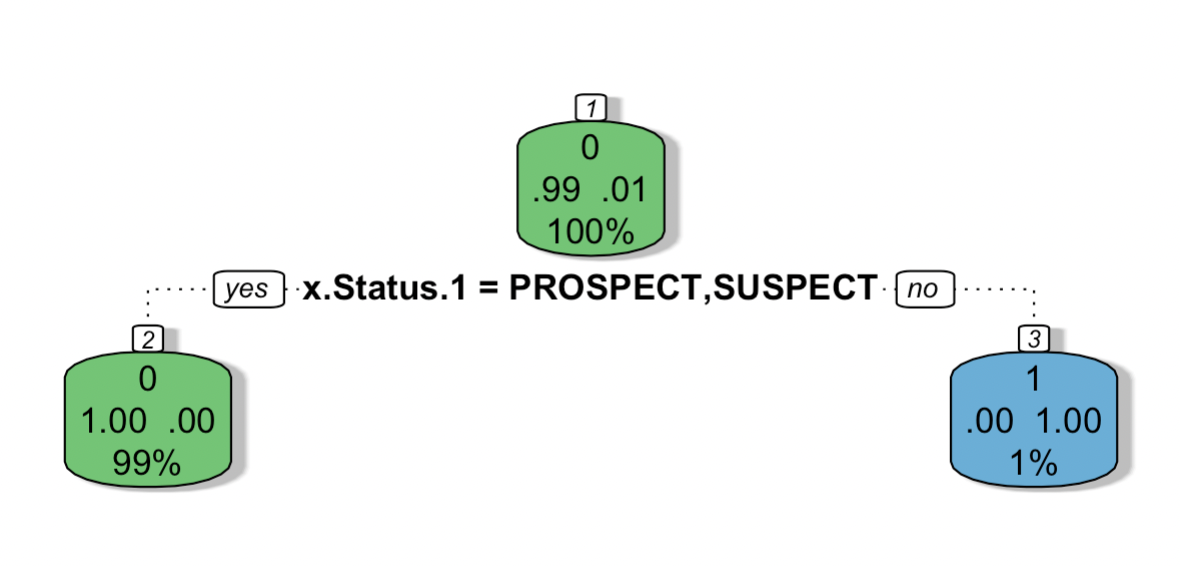Assignment 5: Classifications
Share
Explore#PROBLEM 1: Student Application DataPROBLEM 1: Student Application DataYu Zhu
|

### 1. Run a model to predict if a student will apply to university or not.

`#Question 1: Prediction Model``#Load and clean the data``applicaiton_raw = read.csv("https://raw.githubusercontent.com/jcbonilla/BusinessAnalytics/master/BAData/Univ%20Admissions.csv")``application = read.csv("https://raw.githubusercontent.com/jcbonilla/BusinessAnalytics/master/BAData/Univ%20Admissions.csv",`` header = TRUE, stringsAsFactors=TRUE, na.strings=c('NA',''))``application = na.omit(application)``str(application)`
`#Model Creation``application\$status = ifelse(application\$x.Status.1 == 'SUSPECT', 0, 1)``application\$status = ifelse(application\$x.Status.1 == 'PROSPECT', 0, 1)``application\$status = ifelse(application\$x.Status.1 == 'APPLICANT', 1, 0)``model.app = glm(status ~ x.Gender + x.GPA + `` x.SAT_Score + x.DistancetoCampus_miles +`` x.HouseholdIncome + x.InState + x.Source,`` data = application, family = "binomial")``summary(model.app)`
`#Prediction``app_student_1 = data.frame(x.Gender="Female", x.GPA=3, x.SAT_Score='1050 - 1100', x.DistancetoCampus_miles = 100,`` x.HouseholdIncome = 100000, x.InState = 'Y', x.Source = 'CollegeBoard-Senior_Search')``predict(model.app, app_student_1, type="response")`

`Call:``glm(formula = status ~ x.Gender + x.GPA + x.SAT_Score + x.DistancetoCampus_miles + `` x.HouseholdIncome + x.InState + x.Source, family = "binomial", `` data = application)`
`Deviance Residuals: `` Min 1Q Median 3Q Max ``-1.3884 -0.1892 -0.1395 -0.0984 5.6583 `
`Coefficients:`` Estimate Std. Error z value Pr(>|z|) ``(Intercept) -1.090e+01 9.207e+02 -0.012 0.990555 ``x.GenderMale -3.337e-01 9.749e-02 -3.423 0.000619 ***``x.GPA 3.029e-01 1.661e+00 0.182 0.855256 ``x.SAT_Score1080 - 1350 -2.127e+00 1.520e+00 -1.400 0.161599 ``x.SAT_Score1110 - 1160 -1.298e+01 6.908e+02 -0.019 0.985004 ``x.SAT_Score1170 - 1220 -1.292e+01 5.942e+02 -0.022 0.982652 ``x.SAT_Score1230 - 1280 -1.403e+01 5.994e+02 -0.023 0.981328 ``x.SAT_Score1290 - 1340 -1.240e+01 7.960e+02 -0.016 0.987573 ``x.SAT_Score1350 - 1400 -1.229e+01 9.375e+02 -0.013 0.989544 ``x.SAT_Score1360 - 1530 -3.761e+00 1.673e+00 -2.248 0.024548 * ``x.SAT_Score1410 - 1460 -1.211e+01 9.032e+02 -0.013 0.989306 ``x.SAT_Score930 - 1070 -1.427e+00 1.517e+00 -0.941 0.346877 ``x.SAT_Score930 - 980 -2.867e+00 1.761e+00 -1.628 0.103460 ``x.SAT_Score990 - 1040 -1.244e+01 4.848e+02 -0.026 0.979536 ``x.DistancetoCampus_miles -5.087e-03 9.287e-04 -5.478 4.3e-08 ***``x.HouseholdIncome -1.201e-05 1.442e-06 -8.335 < 2e-16 ***``x.InStateY 4.940e-01 1.272e-01 3.883 0.000103 ***``x.SourceCollegeBoard-Other 8.368e+00 9.207e+02 0.009 0.992748 ``x.SourceCollegeBoard-Senior_Search 9.073e+00 9.207e+02 0.010 0.992137 ``x.SourceNRCCUA-Other -3.291e+00 1.380e+03 -0.002 0.998097 ``x.SourceNRCCUA-Senior_Search -1.694e+00 1.017e+03 -0.002 0.998671 ``x.SourceProspects-Senior_Search 1.084e+01 9.207e+02 0.012 0.990607 ``---``Signif. codes: 0 ‘***’ 0.001 ‘**’ 0.01 ‘*’ 0.05 ‘.’ 0.1 ‘ ’ 1`
`(Dispersion parameter for binomial family taken to be 1)`
` Null deviance: 4919.1 on 34773 degrees of freedom``Residual deviance: 4594.8 on 34752 degrees of freedom``AIC: 4638.8`
`Number of Fisher Scoring iterations: 14`
`> predict(model.app, app_student_1, type="response")`` 1 ``0.1058208 `
By creating a linear regression model and providing a female student example with 3.0 GPA, 1050-1100 SAT Score, and 100 miles distance to campus, the probability for this student to apply is 10.58%.

### 2. Create an appropriate split and validate your model using a ratio of correct predictions vs total predictions.

`#Question 1.2: Split and validate application model``#Create teh training and test data samples``set.seed(100)``split = (.9)``trainingRowIndex1 = sample(1:nrow(application),(split)*nrow(application))``trainingData1 = application[trainingRowIndex1, ]``testData1 = application[-trainingRowIndex1,]``#Develop the model on training data and plot``model.app90 = rpart(status ~. ,data = trainingData1, method = "class")``model.app90``rpart.plot(model.app90)``fancyRpartPlot(model.app90)``#Calculate prediction accuracy``prediction.app = predict(model.app90, testData1, type = "class")``summary(prediction.app)``pd1 = data.frame(actual = testData1\$status, Prediction = prediction.app)``pd1 = table(pd1)``accuracy.app = paste(round((pd1[1,1]+0)/sum(pd1)*100,2), "%")``accuracy.app`

`> model.app90``n= 31296 `
`node), split, n, loss, yval, (yprob)`` * denotes terminal node`
`1) root 31296 424 0 (0.98645194 0.01354806) `` 2) x.Status.1=PROSPECT,SUSPECT 30872 0 0 (1.00000000 0.00000000) *`` 3) x.Status.1=APPLICANT 424 0 1 (0.00000000 1.00000000) *``> rpart.plot(model.app90)``> fancyRpartPlot(model.app90)``> prediction.app = predict(model.app90, testData1, type = "class")``> summary(prediction.app)`` 0 1 ``3439 39 ``> accuracy.app`` "98.88 %"`
Plot of the decision tree:1. From the 10% test data, 3439 of them are under prospect or suspect and 39 of them have applied.
2. The accuracy of data is 98.88%.

### 3. Interpret your model and give actionable recommendations to the marketing department.

Interoperation for the linear regression:
1. Based on the predictors that have been chosen, there are few factors have strong relationship between application status which are gender, SAT score from 1360 to 1530, distance to campus, household income, and in state status.
2. As one unit increase of distance to the campus, the application value would be decreased by 0.0051.
3. As one unit increase of household income, the application value would be decreased by 1.20110^5.
4. If the students is in the state, the application value would be increased by 0.494.
Interoperation for split model:
1. The root node, at the top, shows only 1.0% have applied for this school while 99% are under prospect or suspect.
2. The number above theses proportions indicates that the node is voting (1 = applicant) and the number below indicates the proportion of the population that resides in this node, or impurity.
3. If the student has applied, move right, and if he or she is under prospect or suspect, more left.
Recommendation:
1. Target students who live close to the campus and research on neighborhoods and communities.
2. Target students whose family have relatively low income.
3. Target students who live in the state of the school.
Discussion:
1. Incomplete Data: Based on vacancy of data, more researches about student application could be carried out.
2. Research Range: Similar researches for students who have already been admitted could be carried out for a better explanation.
3. Multiple Factors: Other prediction factors like university reputation, rank, and etc. could be added for further research because there might be some other issues that affect students’ decision.